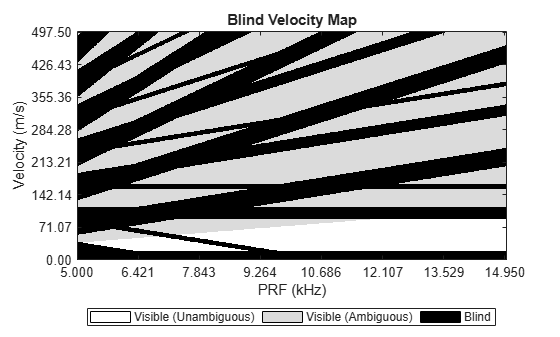# blindvelocitymap

Create blind velocity map for pulsed radar

Since R2023a

## Syntax

``BVM = blindvelocitymap(V,PRF,fc,vmin)``
``BVM = blindvelocitymap(V,PRF,fc,vmin,obstr)``
``[BVM,U] = blindvelocitymap(___)``
``blindvelocitymap(___)``

## Description

````BVM = blindvelocitymap(V,PRF,fc,vmin)` creates a blind velocity map `BVM` for a monostatic pulse-Doppler radar for velocities `V`. The radar transmits at pulse repetition frequencies `PRF`. `fc` represents the radar operating frequency and `vmin` specifies the minimum detectable velocity of the radar.```
````BVM = blindvelocitymap(V,PRF,fc,vmin,obstr)` also specifies obstructions `obstr` in velocity.```

example

````[BVM,U] = blindvelocitymap(___)` also returns the proportion of visible velocities `U`.```
````blindvelocitymap(___)` plots the blind velocity map.```

## Examples

collapse all

A 10 GHz X-band medium PRF radar has a rejection notch to reject slow moving targets with velocities up to 20 m/s. Plot a blind velocity map for pulse repetition frequencies between 5 kHz and 15 kHz, assuming that velocities from 90 m/s to 115 m/s and from 155 m/s to 165 m/s are obstructed due to clutter.

Set up velocities and PRF.

```V = linspace(0,500,1000); PRF = linspace(5e3,15e3,1000);```

Set the radar frequency to 10 GHz.

`fc = 10e9; % Rejection notch half-width (m/s)`

Set the velocity obstructions.

`obstructions = [90 115;155 165];`

Set the rejection notch half-width to 20 meters/sec.

`vmin = 20;`

Create the blind velocity map.

`blindvelocitymap(V,PRF,fc,vmin,obstructions)`## Input Arguments

collapse all

Radar velocities, specified as a length-J real-valued vector of positive values. The function computes the blind velocity map at these velocities Units are in meters/sec.

Example: `[4000,4500,6000]`

Data Types: `double`

Pulse repetition frequencies, specified as a length-N vector of positive values. Units are in Hz.

Example: `[10000,15000]`

Data Types: `double`

Radar waveform carrier frequency, specified as a positive scalar or length-N vector of positive values. If the radar transmits pulses at a fixed carrier frequency, the input `fc` is a scalar. If the radar employs frequency diversity using a different carrier frequency for each PRF value, `fc` is a length-N vector where N is the size of `PRF`.

Example: `3e9`

Data Types: `double`

Minimum detectable velocity, specified as a scalar or length-N real-valued vector. A blind zone due to a non-zero minimum detectable velocity extends from -`vmin` to +`vmin` for all `PRF` values.

• If the radar has the same minimum detectable velocity for all `PRF`s, `vmin` is a scalar and the size of the blind zone is constant for all `PRF` values.

• If the minimum detectable velocity varies with `PRF`, `vmin` is a length-N vector and the size of the blind zone is different for each `PRF`.

Due to ambiguities in velocity, blind zones also appear around integer multiples of the blind speed VB and extend from VB*i - Vmin to VB*i + Vmin, where VB = PRF*c/(2*fc), c is the signal propagation speed, and i is an integer. The input `vmin` can represent the half-width of a rejection notch that is used to reject main lobe clutter and slow moving targets. Units are in meters/sec.

Example: `15.0`

Data Types: `double`

Obstructions in velocity, specified as a Q-by-2 matrix of positive values. The `qth` row specifies a velocity obstruction in the form `[vstartq,vstopq]` where `vstartq` is the start velocity of the `q`Th obstruction and `vstopq` is the stop velocity of the `qth` obstruction, such that `vstopq``vstartq`. Targets moving with the specified obstructed velocities or velocities ambiguous to the specified obstructed velocities are not visible to the radar. Such obstructions can occur due to clutter. Entries in `BVM` that correspond to the obstructed velocities are set to a logical one (`true`). Units are in meters/second.

Example: `[30000;31000]`

Data Types: `double`

## Output Arguments

collapse all

Blind velocity map, returned as a J-by-N logical matrix. The `jth` row of `BVM` represents a velocity cell with the velocity equal to the `jth` entry in `V`. The `nth` column of `BVM` corresponds to the `jth` pulse repetition frequency in `PRF`. If the `jth` velocity in `V` is visible to the radar at the `nth` PRF, `BVM``(j,n)` is a logical zero (`false`), otherwise `BVM``(j,n)` is a logical one (`true`).

Data Types: `logical`

Proportion of visible velocities, returned as a length-N real-valued vector. This argument indicates for each pulse repetition frequency in `PRF` what proportion of velocities in `V` is visible.

## Version History

Introduced in R2023a